Function Repository Resource:

# CausallyInvariantQ

Determine whether a given multiway system evolution is causally invariant

Contributed by: Jonathan Gorard
 ResourceFunction["CausallyInvariantQ"][rules,init,n] yields True if the evolution of the multiway system with the specified rules starting from initial condition init is causally invariant after n steps, and False otherwise.

## Details and Options

A multiway system is causally invariant if the causal networks yielded by each branch of the multiway system are isomorphic as acyclic graphs.
Causal invariance is equivalent to the confluence (or Church–Rosser) property of rewrite systems.
ResourceFunction["CausallyInvariantQ"] determines whether all critical pairs in the multiway system eventually converge back to a common state.
Multiway systems operate on strings, lists and WolframModel systems.
When rules are specified by an explicit association, the following elements can be included:
 "StateEvolutionFunction" the list of successors for a given state "StateEquivalenceFunction" whether two states should be considered equivalent "StateEventFunction" the list of events obtained from a given state "SystemType" system type name "EventSelectionFunction" which events from a given state should be included
The initial condition can consist of a single state or a list of states.

## Examples

### Basic Examples (5)

Determine that a multiway system evolution is causally invariant:

 In:=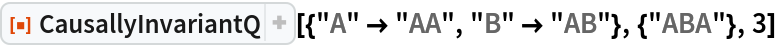Out=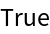In:=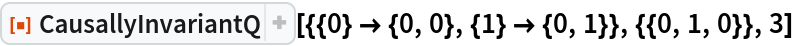Out=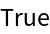Determine that a multiway system evolution is not causally invariant:

 In:=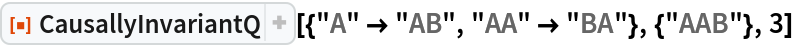Out=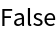In:=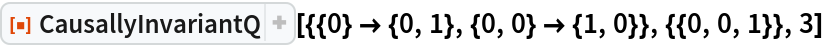Out=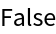Determine that a more complicated multiway system is not causally invariant:

 In:=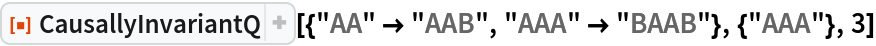Out=In:=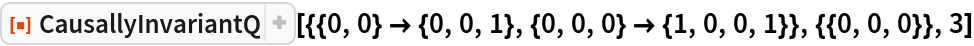Out=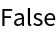By picking a specific updating order, we can force this multiway system to be causally invariant:

 In:=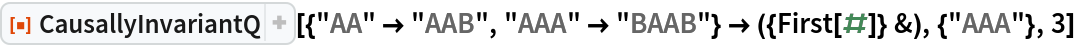Out=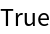In:=Out=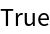CausallyInvariantQ can also determine causal invariance in WolframModel systems:

 In:=Out=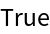In:=Out=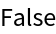### Scope (1)

CausallyInvariantQ returns True if and only if the multiway system yields no unresolved critical pairs:

 In:=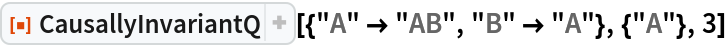Out=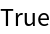In:=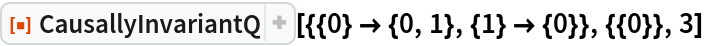Out=Jonathan Gorard

## Version History

• 1.0.0 – 19 November 2019